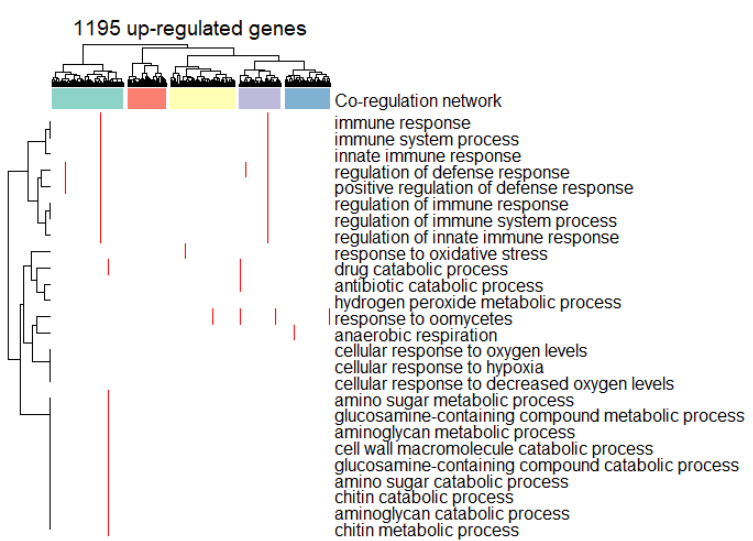Using block annotation with predefined dendrogram
0
0
Entering edit mode
14 months ago
eggrandio ▴ 40

Hi,

I am using ComplexHeatmaps to represent a heatmap data, but I want to cluster the columns by another value, so I am inputting a predefined dendrogram.

I would like to include a Block Annotation to be able to write inside each block.

I am giving a pre-clustered object to cluster_columns and when I try to do cluster_km I get the following message:

Error: You can not perform k-means clustering since you have already specified a clustering object.

I can split the columns using column_split, but then I cannot do block annotation, as my matrix ncols is the number of columns and not the number of clusters.

Error: number of observations in top annotation should be as same as ncol of the matrix.

Basically, I would like to find a way of writing inside each block of a predefined dendrogram.

Code for reference:

#Dimensions of my matrix, and the data I use to make the column dendrogram:
str(mat)
num [1:26, 1:1195] 0 0 0 0 0 0 0 0 0 0 ...
- attr(*, "dimnames")=List of 2
..$: chr [1:26] "response to oxidative stress" "drug catabolic process" "aminoglycan catabolic process" "chitin metabolic process" ... ..$ : chr [1:1195] "AT1G21240" "AT1G09932" "AT2G18690" "AT4G36670" ...

str(MR_genes)
num [1:1195, 1:1195] 0 2.23 2.15 3.54 1.22 ...
- attr(*, "dimnames")=List of 2
..$: Named chr [1:1195] "AT1G21240" "AT1G09932" "AT2G18690" "AT4G36670" ... .. ..- attr(*, "names")= chr [1:1195] "838719" "837526" "816384" "829820" ... ..$ : Named chr [1:1195] "AT1G21240" "AT1G09932" "AT2G18690" "AT4G36670" ...
.. ..- attr(*, "names")= chr [1:1195] "838719" "837526" "816384" "829820" ...

#Clustering of the dendrogram:
clusters=5

dend = as.dendrogram(hclust(as.dist(MR_genes),method="ward.D2"),hang = -1)
cl_num = cutree(dend, k = clusters)

#Building top annotation:
ha_col = brewer.pal(clusters, "Set3")
names(ha_col) = unique(cl_num)
ha_col = list(cl_num=ha_col)

block_ha = HeatmapAnnotation(cl_num = anno_block(gp = gpar(fill = brewer.pal(clusters, "Set3"))),
labels = as.character(c(table(cl_num))))

#Plotting the heatmap with annotation:
mat_col = c("white","red")

Heatmap(mat, col = mat_col,
show_heatmap_legend=F,
cluster_columns = dend,
column_split = clusters,
column_title = top_title,
show_column_names = F,
top_annotation = block_ha,
row_names_max_width = max_text_width(rownames(test)),
clustering_method_rows = "ward.D2")

#Gives the following error:
Error: number of observations in top annotation should be as same as ncol of the matrix.

#If I do this, it works, but I cannot include labels in the blocks:
block_ha = HeatmapAnnotation(cl_num = anno_block(gp = gpar(fill = brewer.pal(clusters, "Set3")))


Here is how my heatmap looks like (without block annotation labels):R complexheatmap dendrogram clustering • 869 views
ADD COMMENT

Login before adding your answer.

Traffic: 1833 users visited in the last hour
Help About
FAQ
Access RSS
API
Stats

Use of this site constitutes acceptance of our User Agreement and Privacy Policy.